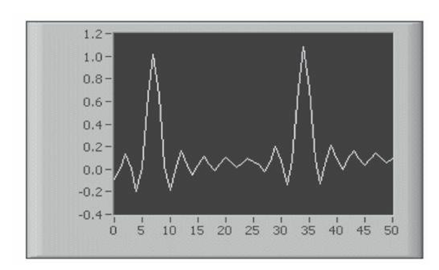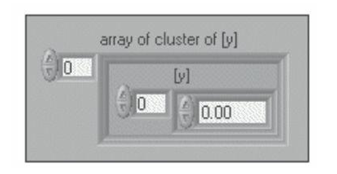# Waveform Graphs In Labview

Rating: 5

11167

LABVIEW includes the WAVEFORM GRAPH and chart to display data typically acquired at a constant rate. The waveform graph displays one or more plots of evenly sampled measurements. The waveform graph plots only single-valued functions, as in y = f (x), with points evenly distributed along the x-axis, such as acquired time-varying waveforms. The below Figure shows an example of a waveform graph. The waveform graph can display plots containing any number of points. The graph also accepts several data types, which minimizes the extent to which you must manipulate data before you display it.

If you would like to Enrich your career with an Android certified professional, then visit Mindmajix - A Global online training platform: “LabVIEW training” Course.This course will help you to achieve excellence in this domain.## Displaying A Single Plot On Waveform Graphs

The waveform graph accepts several data types for single-plot waveform graphs. The graph accepts a single array of values, interprets the data as points on the graph and increments the x index by one starting at x = 0. The graph accepts a CLUSTER of an initial x value, a delta x and an array of y data. The graph also accepts the waveform data type, which carries the data, start time and delta t of a waveform. The waveform graph also accepts the dynamic data type, which is for use with Express VIS. In addition to the data associated with a signal, the dynamic data type includes attributes that provide information about the signal such as the name of the signal or the date and time the data was acquired. Attributes specify how the signal appears on the waveform graph. When the dynamic data type includes a single numeric value, the graph plots the single value and automatically formats the plot legend and x-scale time stamp. When the dynamic data type includes a single channel, the graph plots the whole waveform and automatically formats the plot legend and x-scale time stamp.## Displaying Multiple Plots On Waveform Graphs

The waveform graph accepts several data types for displaying multiple plots. The waveform graph accepts a 2D ARRAY of values, where each row of the array is a single plot. The graph interprets the data as points on the graph and increments the x index by one, starting at x = 0. Wire a 2D array data type to the graph, right-click the graph, and select Transpose Array from the shortcut menu to handle each column of the array as a plot. This is particularly useful when you sample multiple channels from a DAQ device because the device can return the data as 2D arrays with each channel stored as a separate column. The waveform graph also accepts a cluster of an initial x value, a delta x value, and a 2D array of y data. The graph interprets the y data as points on the graph and increments the x index by delta x, starting at the initial x value. This data type is useful for displaying multiple signals that are sampled at the same regular rate. The waveform graph accepts a plot array where the array contains clusters. Each cluster contains a 1D array that contains the y data. The inner array describes the points in a plot, and the outer array has one cluster for each plot. The front panel below Figure shows the array of the cluster.Use a plot array instead of a 2D array if the number of elements in each plot is different. For example, when you sample data from several channels using different time amounts from each channel, use this data structure instead of a 2D array because each row of a 2D array must have the same number of elements. The number of elements in the interior arrays of an array of clusters can vary. The waveform graph accepts a cluster of an initial x value, a delta x value, an array that contains clusters. Each cluster contains a 1D ARRAY that contains the y data. You use the Bundle function to bundle the arrays into clusters and you use the Build Array function to build the resulting clusters into an array. You also can use the Build Cluster Array function which creates arrays of clusters that contain the inputs you specify.

The waveform graph accepts an array of clusters of an x value, a delta x value, and an array of y data. This is the most general of the multiple-plot waveform graph data types because you can indicate a unique starting point and increment for the x-scale of each plot. The waveform graph also accepts the dynamic data type, which is for use with Express VIs. In addition to the data associated with a signal, the dynamic data type includes attributes that provide information about the signal, such as the name of the signal or the date and time the data was acquired. Attributes specify how the signal appears on the waveform graph. When the dynamic data type includes multiple channels, the graph displays a plot for each channel and automatically formats the plot legend and x-scale time stamp.

Check Out LabVIEW TutorialsCourse Schedule
NameDates
LabVIEW TrainingJan 31 to Feb 15
LabVIEW TrainingFeb 04 to Feb 19
LabVIEW TrainingFeb 07 to Feb 22
LabVIEW TrainingFeb 11 to Feb 26
Last updated: 29 January 2023